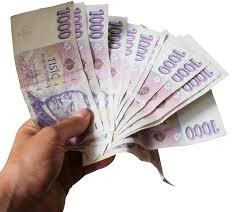# Money spending

Albert and Peter have an amount of money. If Albert spent $6 and Peter did not spend any, then the ratio of Albert's money to Peter's money is 1:3 . If Peter spent$6 and Albert did not spend any, the ratio of Albert's money to Peter's money is 3:7.How much money does each of them has?

A =  36 USD
P =  90 USD

### Step-by-step explanation:Did you find an error or inaccuracy? Feel free to write us. Thank you!

Tips for related online calculators
Need help calculating sum, simplifying, or multiplying fractions? Try our fraction calculator.
Check out our ratio calculator.
Do you have a system of equations and looking for calculator system of linear equations?